# Incenter Theorem – Definition, Conditions and Examples

The incenter theorem shows that the angle bisectors dividing the triangle’s vertices are concurrent. This theorem establishes the properties and formula of incenters, inradius, and even incircles. These properties and theorem open a wide range of applications and other properties of triangles.

The incenter theorem states that the incenter (intersection of the triangle’s angle bisector) is equidistant from all three sides of the triangle.

This article covers the fundamentals of the incenter theorem and lays down the properties involving the incenter and the process of locating the incenter depending on the given components of the triangle.

## What Is the Incenter Theorem?

The incenter theorem is a theorem stating that the incenter is equidistant from the angle bisectors’ corresponding sides of the triangle. The angle bisectors of the triangle intersect at one point inside the triangle and this point is called the incenter.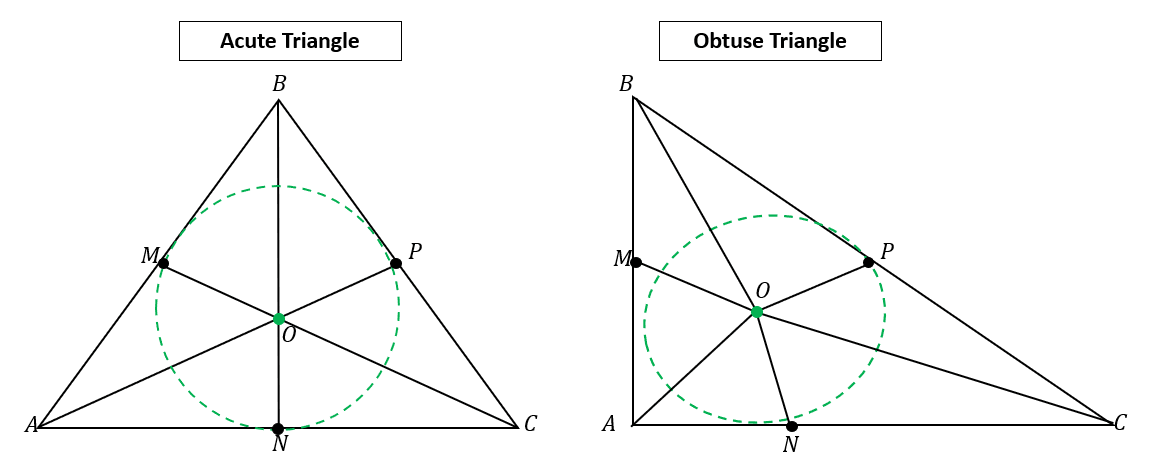Take a look at the two triangles shown above, the point $O$, where three of the angle bisectors meet, is what we call the incenter. The incenter theorem establishes the fact that the incenter $O$ shares the same distance from the points on the triangle’s sides: $M$, $N$, and $P$.

 Incenter TheoremThis means that when $\overline{AO}$, $\overline{BO}$, and $\overline{CO}$ are the angle bisectors of the triangle $\Delta ABC$, the following are equidistant:\begin{aligned}\boldsymbol{\overline{MO} = \overline{NO} = \overline{PO}}\end{aligned}

It has been established that the incenter is equidistant from the points lying on each side of the triangle. This means that when a circle is inscribed within the triangle, the radius will be the same distance of the incenter from the side, making it the center of the inscribed circle. We call the circle satisfying this condition an incircle.

Aside from the equal distances shared between the incenter and the triangle’s sides, the incenter of the triangle also exhibits interesting properties. Thanks to the incenter theorem, these properties can be established as well.

### Properties of the Incenter of a Triangle

The properties of the triangle’s incenter include the relationship shared between the triangle’s angles as well as how the perimeters behave when given the incenter.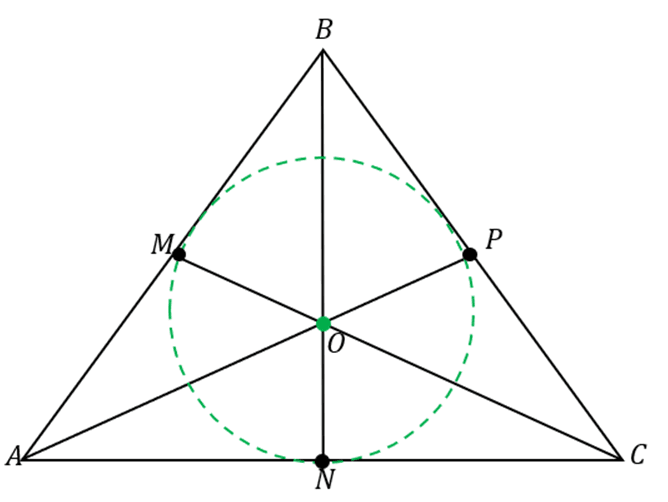Refer to the triangle shown above as a guide when studying the properties shown below.

• Property 1: Given the triangle’s incenter, the line passing through it from the vertices of the triangle are angle bisectors. This means that the smaller angles formed by these lines are equal to each other.

\begin{aligned}\angle BAO &= \angle CAO\\\angle BCO&= \angle ACO\\\angle ABO &= \angle CBO\end{aligned}

• Property 2: Given the triangle’s incenter, the adjacent sides forming the included angle of the bisector are equal. This applies to all pairs of segments, so for $\Delta ABC$ with an incenter of $O$, we have the following:

\begin{aligned}\overline{AM} &= \overline{AN}\\\overline{CN} &= \overline{CP}\\\overline{BM} &= \overline{BP}\end{aligned}

• Property 3: As an extension of the incenter theorem, when an incircle is constructed in a circle, the radius’ measure can be established as shown below.

\begin{aligned}\overline{OM}= \overline{ON}= \overline{OP}\end{aligned}

These line segments are also called the inradii of the circle. The fourth property deals with the semi-perimeter of the triangle, and as a refresher, the semi-perimeter of a triangle is simply half the perimeter of the triangle.

\begin{aligned}\Delta ABC_{\text{Semiperimeter}} &= \dfrac{\overline{AB}+ \overline{BC} + \overline{AC}}{2}\end{aligned}

• Property 4: Given the semi-perimeter of the triangle, $s$, and the inradius of the triangle, $r$, the area of the triangle is equal to the product of the perimeter and the inradius.

\begin{aligned}S&= \dfrac{\overline{AB}+ \overline{BC} + \overline{AC}}{2}\\A_{\Delta ABC} &= S \cdot r\end{aligned}

After learning about the four important properties of incenter, it’s time to apply the incenter theorem and these properties to learn how to locate incenters. The next section covers the important processes of locating and constructing incenters.

## How To Find the Incenter of a Triangle

There are three ways to find the incenter of the triangle: using the algebraic formula for coordinates, measuring the inradius, and graphically constructing the incenter. When finding the incenter of a triangle, use the fact that incenters are points where the angle bisectors intersect.

1. If a triangle is located on a coordinate system, apply the incenter formula to find the coordinates of the triangle’s incenter.
2. The incenter can also be located graphically by constructing the angle bisectors of the triangle.
3. Calculate the inradius and construct inradii from each of the vertices to locate the incenter of the triangle.

This section covers the three methods to highlight the instances when each method is most helpful given the situation.

### Finding the Incenter in a Coordinate Plane

To find the incenter of a triangle graphed on an $xy$-plane, use the coordinates of the triangle’s vertices then apply the incenter formula to find the incenter’s formula.

\begin{aligned}\color{DarkOrange}\textbf{Incenter Formula}\phantom{xxxxxx}\\\left(\dfrac{ax_1 + ax_2 + ax_3}{a + b+ c}, \dfrac{ay_1 + ay_2 + ax_3}{a + b+ c} \right)\end{aligned}

Let’s break down the formula and learn how to apply this by taking a look at the triangle shown below.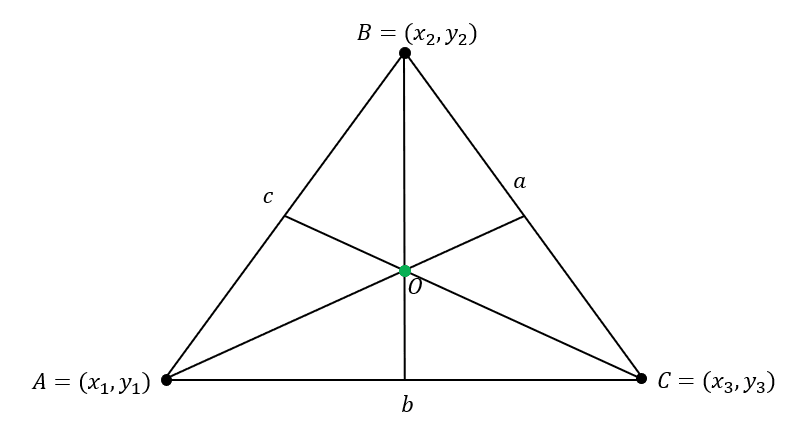Suppose that $\Delta ABC$ has the following coordinates: $A = (x_1, y_1)$, $B = (x_2, y_2)$, and $C = (x_3, y_3)$. In addition, the triangle’s sides have the following lengths:

\begin{aligned}\overline{AB} &= c\\\overline{BC} &= a\\\overline{AC} &= b\end{aligned}

Find the incenter’s coordinate by multiplying the lengths of $\Delta ABC$ to the corresponding coordinate of the vertices then combining the $x$ and $y$-coordinates’ values.

\begin{aligned}\text{Incenter}_{(x, y)} &= \left(\dfrac{ax_1 + bx_2 +cx_3}{a + b + c}, \dfrac{ay_1 + by_2 +cy_3}{a + b + c}\right)\end{aligned}

If the side’s lengths are not given, use the distance formula, $d =\sqrt{(x_2 – x_1)^2 + (y_2 – y_1)^2 }$, to calculate the length of $a$, $b$, and $c$.

### Finding the Incenter by Constructing Angle Bisectors

When given the triangle, it is also possible to find the incenter by constructing the three angle bisectors of the triangle’s vertices. Recall that the angle bisectors divide the angles into two congruent angles each.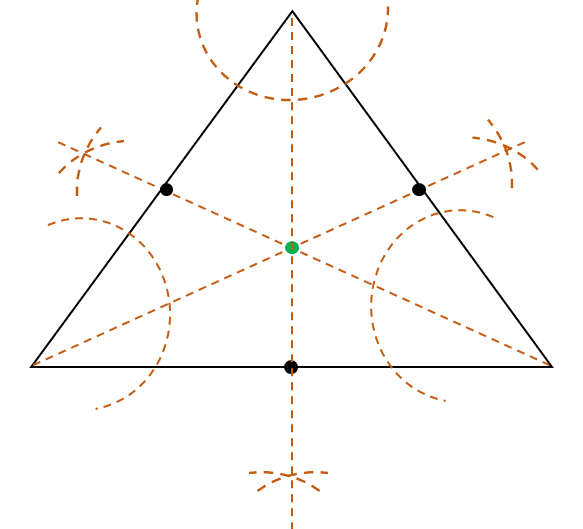Divide each angle measure of the three vertices then construct the three angle bisectors. These three angle bisectors are concurrent, which means that they will meet at one point. Locate this point to find the incenter’s position.

### Finding the Incenter Using the Inradius

It is also possible to find the incenter using the inradius of the triangle. This method is helpful especially when the incircle and the lengths of the triangle’s sides are given. Calculate the measure of the inradius using the triangle’s side lengths and semi-perimeter.

\begin{aligned}S&= \dfrac{a + b + c}{2}\\r&= \sqrt{\dfrac{(S – a)(S – b)(S – c)}{S}}\end{aligned}

In this formula, $S$ represents the semi-perimeter of the triangle, whereas $a$, $b$, and $c$ are the side lengths of the triangle.

Once the inradius’ measure is given, plot the incenter from the incircle going $r$ units towards the center. This presents the position of the incenter.

Now that we’ve learned the different ways to find the incenter of a triangle, it’s time to practice different problems involving the incenter and the incenter theorem. When ready, head on over to the section below!

### Example 1

The triangle $\Delta ABC$ has the following angle bisectors: $\overline{MC}$, $\overline{AP}$, and $\overline{BN}$. These angle bisectors meet at point, $O$. Suppose that $\overline{MO} = (4x + 17)$ cm and $\overline{OP} = (6x – 19)$ cm, what is the measure of $\overline{MO}$?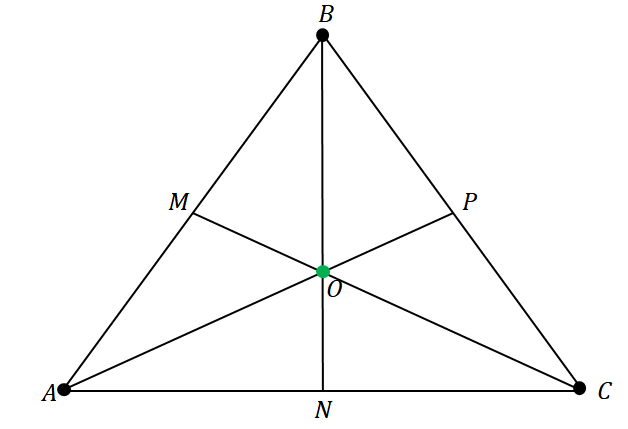### Solution

The three angle bisectors meet the point $O$, so the point is the incenter of the triangle $\Delta ABC$. According to the incenter theorem, the incenter is equidistant from all three sides of the triangle.

\begin{aligned}\overline{MO} = \overline{ON} = \overline{OP}\end{aligned}

Since $\overline{MO} = (4x + 17)$ cm and $\overline{OP} = (6x – 19)$ cm, equate these two expressions to solve for $x$.

\begin{aligned}\overline{MO} &= \overline{OP}\\ 4x + 17&= 6x – 19\\ 4x – 6x &= -19 – 17\\-2x &= -36\\x &= 18\end{aligned}

Substitute the value of $x = 18$ into the expression for the length of $\overline{MO}$.

\begin{aligned}\overline{MO} &= 4x + 17\\ &= 4(18) + 17\\&= 89\end{aligned}

This means that length of $\overline{MO}$ is equal to $89$ cm.

### Example 2

The three points $A = (10, 20)$, $B = (-10, 0)$, and $C = (10, 0)$ are the three vertices of the triangle $\Delta ABC$ graphed on the $xy$-plane. What are the coordinates of the triangle’s incenter?

### Solution

Plot the three points on the $xy$-plane then use these as vertices to construct the triangle $\Delta ABC$. Now, find the lengths of the triangle’s three sides.

• $\overline{AC}$ and $\overline{BC}$’ lengths are easy to find since they are vertical and horizontal lines, respectively.

\begin{aligned}\overline{AC} = \overline{BC} = 20\end{aligned}

• Use the distance formula, $d= \sqrt{(x_2 – x_1)^2 + (y_2 – y_1)^2}$, to find the length of $\overline{AB}$.

\begin{aligned}\overline{AB} &= \sqrt{(10 – -10)^2 + (20 -0)^2}\\&= 20\sqrt{2}\end{aligned}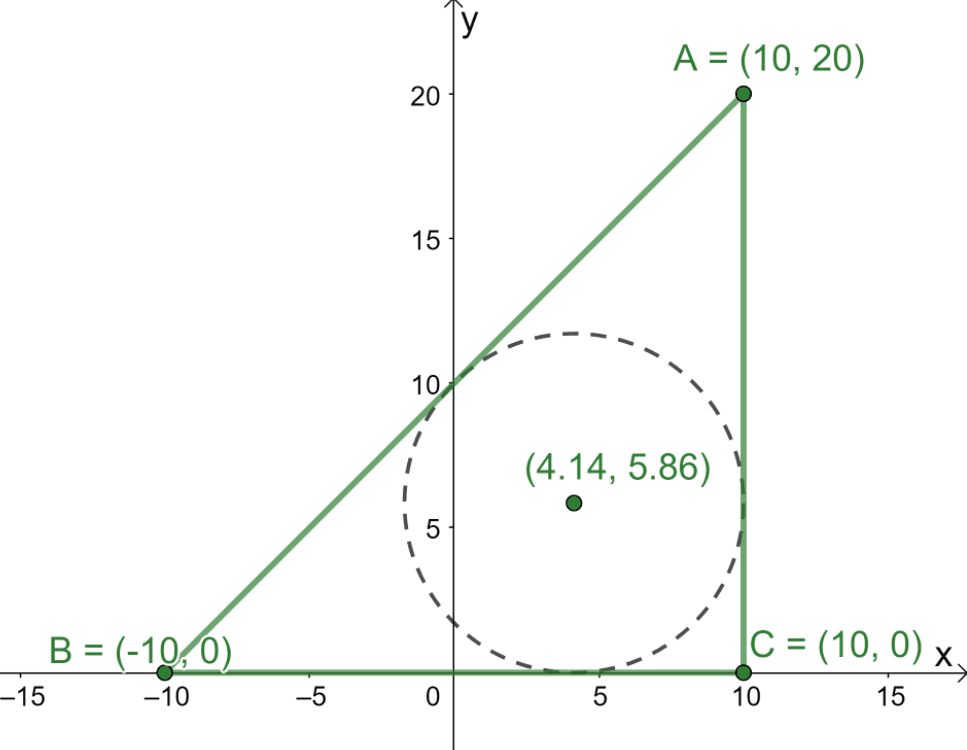Now that we have the lengths of $\Delta ABC$’s three sides, use the incenter formula to find the coordinates of the triangle’s incenter.

\begin{aligned}\text{Incenter}_{(x, y)} &= \left(\dfrac{ax_1 + bx_2 +cx_3}{a + b + c}, \dfrac{ay_1 + by_2 +cy_3}{a + b + c}\right)\\\end{aligned}

Substitute the following values into the incenter formula: $a = 20$, $b = 20$, $c = 20\sqrt{2}$, $(x_1, y_1) = (10, 20)$, $(x_2, y_2) = (-10, 0)$, and $(x_3, y_3) = (10, 0)$.

\begin{aligned}\text{Incenter}_{(x, y)} &= \left(\dfrac{20 \cdot 10 + 20 \cdot -10 +20\sqrt{2} \cdot 10}{20 + 20 + 20\sqrt{2}}, \dfrac{20 \cdot 20 + 20 \cdot 0 +20\sqrt{2} \cdot 0}{20 + 20 + 20\sqrt{2}}\right)\\&= \left(\dfrac{200\sqrt{2}}{30 + 20\sqrt{2}},\dfrac{400}{40 + 20\sqrt{2}}\right)\\&\approx (4.14, 5.86)\end{aligned}

From this, we now know that the incenter is located approximately at the point $(4.14, 5.86)$.

### Practice Questions

1. The triangle $\Delta ABC$ has the following angle bisectors: $\overline{MC}$, $\overline{AP}$, and $\overline{BN}$. These angle bisectors meet at point $O$. Suppose that $\overline{MO} = (6x – 23)$ ft and $\overline{OP} = (4x + 29)$ ft, what is the length of $\overline{OP}$?A. $\overline{OP}$ is $123$ units long.
B. $\overline{OP}$ is $133$ units long.
C. $\overline{OP}$ is $143$ units long.
D. $\overline{OP}$ is $153$ units long.

2. The three points $A = (30, 40)$, $B = (-10, 0)$, and $C = (30, 0)$, are the three vertices of the triangle $\Delta ABC$ graphed on the $xy$-plane. What are the coordinates of the triangle’s incenter?

A. $(17.18,10.62)$
B. $(18.18,11.62)$
C. $(18.28,11.72)$
D. $(19.28,12.72)$